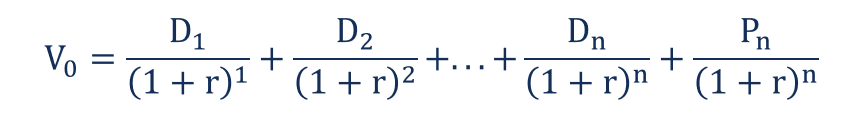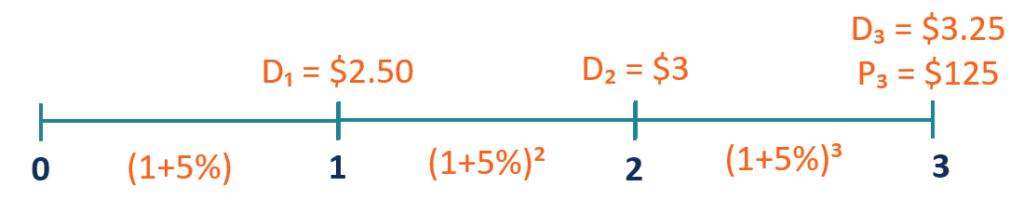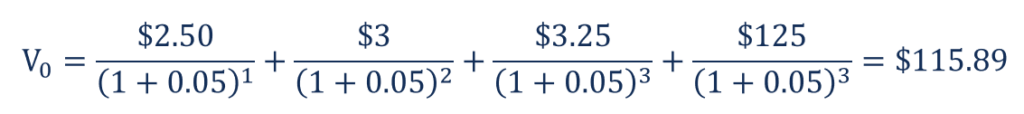# Multiple-Period Dividend Discount Model

How to calculate the intrinsic value of a stock that will be held for a finite number of periods

## What is the Multiple-Period Dividend Discount Model?

A multiple-period dividend discount model is a variation of the dividend discount model. It is often used in situations when an investor is expecting to buy a stock and hold it for a finite number of periods and sell the stock at the end of the holding period.

Similar to the general dividend discount model, the multiple-period model is based on the assumption that the intrinsic value of a stock equals the sum of all future cash flows discounted back to their present values.In such a scenario, an investor expects to hold the stock for the multiple periods. Thus, the future cash flows from the stock will include several dividend payments, as well as the expected selling price of the stock.

Subsequently, the current intrinsic value of a stock may be calculated by finding the sum of the future dividend payments and the anticipated selling price discounted back to their present values.

### Formula for the Multiple-Period Dividend Discount Model

The mathematic formula that helps to calculate the fair value of a stock using the multiple-period dividend discount formula is given below:Where:

• V– the current fair value of a stock
• D– the dividend payment in the nth period from now
• P– the stock price in the nth period from now
• r – the estimated cost of equity capital

### Example of Multiple-Period Dividend Discount Model

You are an investment analyst. Your client asked you to assess the viability of the investment in ABC Corp. The client expects to hold the investment for three years and sell it at the end of the holding period (end of the third year).

You’ve forecasted that ABC Corp. will pay dividends of \$2.50 in the first year, \$3 in the second year, and \$3.25 in the third year. You expect that at the end of the third year, the selling price of the company’s stock will be \$125 per share. The estimated cost of capital is 5%. The current stock price is \$110 per share.In order to assess the viability of the investment, you should determine the intrinsic value of the company’s stock. It can be found using the multiple-period dividend discount model. By inputting the known variables into the formula, the intrinsic stock value can be calculated in the following way:The intrinsic value of the company’s stock is \$115.89, which is more than its current stock price (\$110). Therefore, we can say that the stock is currently undervalued.

CFI is the official provider of the global Financial Modeling & Valuation Analyst (FMVA)™ certification program, designed to help anyone become a world-class financial analyst. To keep advancing your career, the additional resources below will be useful:

• Ex-Dividend Date
• FCFF vs FCFE vs Dividends
• One-Period Dividend Discount Model
• Valuation Methods

### Valuation Techniques

Learn the most important valuation techniques in CFI’s Business Valuation course!

Step by step instruction on how the professionals on Wall Street value a company.

Learn valuation the easy way with templates and step by step instruction!